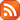Subscribe by RSS

# Atiyah-Singer Index Seminar

Tue., Mar. 8, 2016 10:00 a.m.

Location: RIC 215

• Speaker:  Asghar Ghorbanpour
• Title: Classical index theorems I: Gauss-Bonnet Theorem
• Abstract:

Despite of very geometric form of the Gauss-Bonnet theorem, it turns out that the theorem is indeed the index theorem of a geometric elliptic differential operator that can be defined on Riemannian manifolds. The goals of this series of lectures are to see different versions of this theorem, how it has evolved to be an index theorem and what is the operator whose index is computed by this theorem. Later on, we will show that the Gauss-Bonnet can be proved as a corollary of the Atiyah-Singer index theorem.